# 10. (a) Compute the second-order Taylor series approximation to the function f(x, y, z)e(z-1)² cos(y).(b) Using the approximation found in (a) to approximate the value of f S Sp f(x, y, z, )dxdydz,where D is the cube 0 < x, y, z < 3.

Question
14 views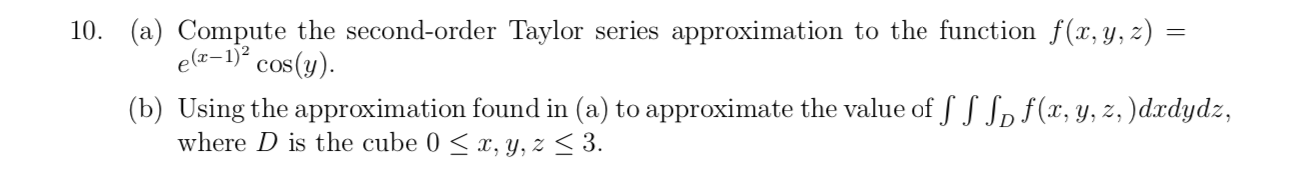help_outlineImage Transcriptionclose10. (a) Compute the second-order Taylor series approximation to the function f(x, y, z) e(z-1)² cos(y). (b) Using the approximation found in (a) to approximate the value of f S Sp f(x, y, z, )dxdydz, where D is the cube 0 < x, y, z < 3. fullscreen
check_circle

Step 1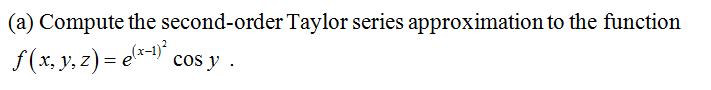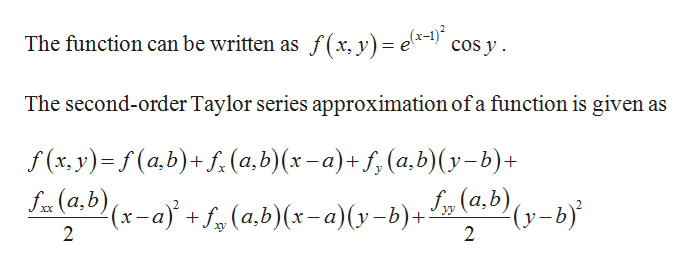help_outlineImage TranscriptioncloseThe function can be written as f ( x, y) = e*-!) cos y . The second-order Taylor series approximation of a function is given as f (x, y)= f (a,b)+ f, (a,b)(x-a)+ f,(a,b)(y-b)+ S„ (a.b) (y-b) fx (a,b) (x-a)} + f, (a,b)(x-a)(y-b)+ 2 fullscreen
Step 2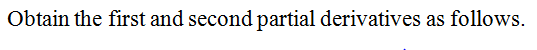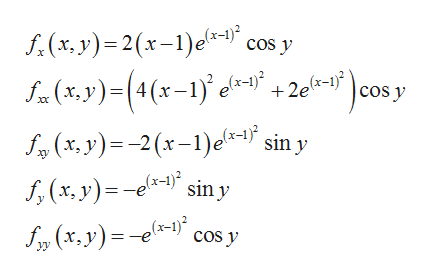help_outlineImage Transcriptionclosef, (x, y)= 2(x-1)ela-1)" cos y Sa (x,y)=(4(x-1) e1 + 20*-s° )cos y fx (x,y (x-1)° +2e f„ (x, y) = -2(x-1)e=- sin y f, (x. y) = -e*- sin y S, (x,y)=-e*-1)" cos y elx=1)² fullscreen
Step 3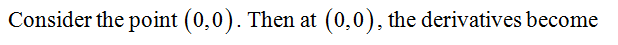...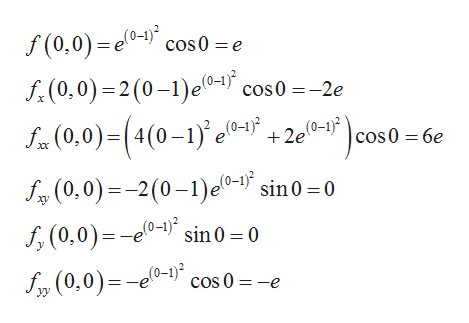help_outlineImage Transcriptionclosef (0,0) = e0-) cos0 = e f.(0,0) =2(0-1)eº-1) cos0 =-2e S- (0,0)=(4(0–1)' e0-³° +2e (0-1)? +2e(0-1}? (0-1)² cos0 = 6e f, (0.0) =-2(0–1)e0-" sin0 =0 f, (0,0)=-e0-1)*, sin 0 = 0 fm (0,0)=-eº- cos 0 = -e yy fullscreen

### Want to see the full answer?

See Solution

#### Want to see this answer and more?

Solutions are written by subject experts who are available 24/7. Questions are typically answered within 1 hour.*

See Solution
*Response times may vary by subject and question.
Tagged in